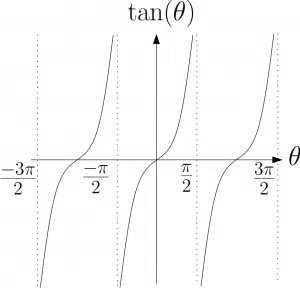# Trigonometry (study of triangles) in A-Level Maths

## Trigonometry

Trigonometry is the study of triangles and many trigonometric functions come from studying triangles. You have seen some of these functions before. Namely the sin, cos and tan functions at GCSE.  At A-Level, we study these and other trigonometric functions, their equations and also their identities. We also look at approximations in trigonometry as well as measuring angles and sketching curves using radians as opposed to degrees.Firstly, basic trigonometric graphs, equations and identities are explored in the first year of A-Level Maths as well as non-right angled triangles. Then, in the second year, students will also look at radians, arcs & sectors, small angle approximations, the sec/cosec/cotan functions and inverse trigonometry.